## ISIS 2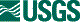ISIS Documentation

# ISIS and Image Processing Terms and Definitions

Home

## A Priori

The term, "A Priori", refers to the initial or original value of a pixel coordinate or geographic location within a control network. Applications such as pointreg or jigsaw refer to the a priori values for an input pixel coordinate (pointreg) or geographic location (jigsaw) in an input control network.

The term, Adjusted, refers to the output pixel coordinate or output geographic location within a control network. Applications such as pointreg or jigsaw report and update the "Adjusted" output pixel coordinate (pointreg) or geographic location (jigsaw) in the output control network.

## Albedo

Reflectivity of a surface or particle. Commonly in I/F units for radiometrically calibrated data.

## Azimuth

A clockwise angle from a point of origin to a point of interest.

The azimuth is measured in a positive clockwise direction from a reference line. The reference line is formed by a line drawn horizontally from the origin point to the right side of the image (3 o'clock reference if the image is viewed as a clock face) Azimuth can also be referred to as Image Azimuth.

## Band

A 3rd dimensional layer of pixels in an image, typically representing spectral information. The number of bands indicates the depth of an image in terms of these layers.

## Bit

Short for binary digit, which in a computer is the smallest unit of storage. Bits are either "0" or "1".

## Bit Type

Refers to how many bits there are per single meaningful value in an image cube file.

## Body Fixed Coordinate

A planetary coordinate system where the coordinates are not time varying.

For a body-fixed reference frame, ISIS refers to the NAIF planet kernel (PCK) for high-accuracy orientation (rotation) data for any solar system target body. This kernel includes location of the pole, prime meridian, axis directions of a body-fixed, body-centered reference frame and spin rate.

## Body-Fixed Coordinate System

A body-fixed coordinate system is used to determine positions and directions of objects and vectors with respect to a target body. The origin is at the center of mass for the target and the axes rotate and move through space with the body. These systems are non-inertial, meaning the velocity of the origin is non-constant. Many body-fixed coordinate systems, or reference frames, are defined in NAIF PCK files.

## Byte

Short for binary term. A byte is a collection of computer bits. On most modern computers, a byte is a group of eight bits. Typically, computers handle and store information in binary (base-2), and a group of eight bits represents an eight digit binary number.

Within the ISIS environment, "Byte" refers to how many bits there are per single meaningful value in an image cube file. The Byte information for an image cube file is stored on the image cube labels in Group=Pixels, where the keywords Type and ByteOrder describe the byte format.

## Camera Coordinate System

A camera coordinate system is used to determine positions and directions of objects and vectors with respect to a sensor (i.e. camera). The origin is at the center of the sensor and the axes rotate and move through space with the sensor. These systems are non-inertial, meaning the velocity of the origin is non-constant. The location of the center of a spacecraft and its instruments at a given time are defined in NAIF SPK files. Generally, one or more frames are associated with a particular instrument.

## Control Island

Ideally a control network will represent a single island of a list of overlapping images all tied together through a network of control points and image measures (Refer to Control Measures).

Multiple islands can be identified within a single network if groups of images are isolated from one another due to no overlap or the absence of possible image measures.

## Control Measure

The sample and line pixel coordinate (measurement) within a single image cube that has been measured and associated with a Control Point and other overlapping image cubes.

## Control Network

A network of Control Points. Within the ISIS environment, the control network file is binary file. The network contains multiple fields of information for control points and measures collected between any number of image cubes.

## Control Point

One or more measurements (image coordinates) that identify the same feature or location in different overlapping images.

## Cube

A cube is a 3-dimensional image with axis: samples, lines, and bands. The physical dimensions of a cube are called the number of samples (NS), number of lines (NL), and number of bands (NB).

Typically, the sample and line dimensions are used to represent spatial information while the band dimension represents spectral information.

## Declination

Declination (Dec) is one of two angles of the North Pole of a target body as a function of time.

For more information, refer to Euler Angles, Right Ascension and Prime Meridian

## Detector Resolution

The ground distance in meters from the left edge to the right edge of a detector. If the lines and samples are not being summed and averaged, this value is equal to the pixel resolution. The formula for estimating this value works well if the image is taken near nadir, but the accuracy falls off rapidly the farther off-nadir the image is taken. For an estimate that handles off-nadir images with higher accuracy, one should use oblique detector resolution.

## Digital Number

The numeric value of a single pixel in an image. The value may represent almost any unit. For example: reflectance (I/F), radiance, elevation, or radius. Digital Numbers (DNs) can be discrete integers or floating point values.

## Elevation

The height above or below a fixed point on the surface of a body.

## Emission Angle

The emission angle (EMA) is the angle between the spacecraft and a vector drawn perpendicular to the planet's surface (surface normal). Using SPICE, ISIS applications compute this angle from the ellipsoid.

Emission Angle is in degrees. The valid range is between 0 and 90.

## Emissivity

A measure describing a substance's ability to absorb and radiate electromagnetic energy.

## Ephemeris Time

Ephemeris Time (ET) as defined by NAIF (Toolkit used by ISIS), is the uniform scale represented by the independent variable in the differential equations that describe the motions of the planets, sun and moon. Ephemeris time is described as a count of ephemeris seconds past the ephemeris reference epoch (J2000).

The basic spatial reference system for SPICE is the J2000 system. This is an inertial reference frame in which the equations of motion for the solar system may be integrated. This reference frame is specified by the orientation of the earth's mean equator and equinox at a particular ephoch...the J2000 epoch. This epoch is Greenwich noon on January 1 2000 Barycentric Dynamical Time.

Ephemeris Time is in seconds.

The distance from the geometric center of an ellipsoid to its equator.

## Euler Angles

All the NAIF orientation models use three Euler angles to describe body orientation. The Euler angles describe the orientation of the coordinate axes of the 'body equator and prime meridian' system with respect to the J2000 system.

For more information, refer to Right Ascension (RA), Declination (Dec) and Prime Meridian (W)

## Ground Azimuth

A clockwise angle between one reference point and another reference point. Each pixel location has a latitude and longitude associated with it (ground point). The only information required to calculate a ground azimuth is to form a spherical triangle from three points on the planet (ground point, North Pole, and point of interest). The point of interest is often the sub-spacecraft or sub-solar point. Spherical trigonometry is then used to calculate the angle.

Ground Azimuth has nothing to do with the image, meaning it does not rely on camera geometry for its calculation.

## HIS

HIS [High Instrument Saturation] is an instrument special pixel value that is tracked and reported to the user. HIS is set during the ingestion of the image data into ISIS. The input image sensor acquired a value too high to be measured accurately.

HIS represents "Oversaturated". HIS is set in 16-and 32-bit type image data. For 8-bit image data, HIS = NULL.

## HRS

HRS [High Representation Saturation] is a representation special pixel value that is tracked and reported to the user. HRS is set when an ISIS application causes the output DN pixel to fall 'above' the possible range of valid values.

HRS represents "Oversaturated". HRS is set in all 8, 16, 32-bit type image data.

## I/F

Irradiance/SolarFlux. Unit of DN values for a reference distance of target body from the Sun (5.2 AU). The valid values range between 0.0 and 1.0.

I/F is often a default or choice of output DN unit of the mission specific radiometric calibration applications.

## IAU

The International Astronomical Union

## Incidence Angle

The incidence angle (INC) is the angle between the sun and the surface normal. ISIS applications use SPICE to compute this angle from the ellipsoid or the elevation model (e.g., local slopes) at every pixel.

Incidence Angle is in degrees. The valid range is between 0 - 90. Data can be illuminated beyond the 90 degrees in the deep terminator region. While this is valid, most photometric functions will not normalize beyond 90 degrees.

Incidence Angle is in degrees.

## J2000 Coordinate System

The J2000 coordinate system (also known as EME2000) is based on the earth mean equator and dynamical equinox at midnight January 1, 2000. The origin is at the solar system barycenter. This system is inertial, since it does not rotate with respect to stars and the origin is not accelerating (i.e it has a constant velocity). This coordinate system is the root reference for NAIF's SPICE files and software.

## LIS

LIS [Low Instrument Saturation] is an instrument special pixel value that is tracked and reported to the user. LIS is set during the ingestion of the image data into ISIS. The input image sensor acquired a value too low to be measured accurately.

LIS represents "Undersaturated". LIS is set in 16-and 32-bit type image data. For 8-bit, LIS = NULL.

## LRS

LRS [Low Representation Saturation] is a representation special pixel value that is tracked and reported to the user. LRS is set when an ISIS application causes the output DN pixel to fall 'below' the possible range of valid values.

LRS represents "Undersaturated". LRS is set in 16-and 32-bit type image data. For output 8-bit, LRS = NULL.

## Latitude

Latitude represents a geographic position and is measured from the equator, with positive longitudes going North and negative values going South.

Latitude is in degrees.

Refer to the definition for Latitude Type for more information within the ISIS environment.

## Latitude Type

Latitudes can be represented either in Planetographic or Planetocentric form. Both latitudes are equivalent on a sphere (i.e., the equatorial radius is equal to the polar radius); however they differ on an ellipsoid body (e.g., Mars, Earth).

The default for Latitude Type in ISIS is Planetocentric. Latitude Type will affect the computed values for latitude range (Minimum and Maximum Latitude) and Center latitude. Refer to the definitions for Planetocentric Latitude and Planetographic Latitude.

Level2 map projections such as Sinusoidal, Simple Cylindrical, and Equirectangular will differ depending on Latitude Type for non-spherical bodies. Other map projections are not affected.

## Level0

Level0 is a standard cartographic processing phase that describes an image has been ingested into ISIS (PDS EDR) and will often have SPICE information loaded on the ISIS cube labels (spiceinit). Level0 image cubes are often the input to mission specific radiometric calibration applications if supported in ISIS.

Level0 implies a 'non-projected', raw image cube ('camera space').

## Level1

Level1 is a standard cartographic processing phase that describes an image has been radiometrically calibrated (PDS CDR or the ISIS output of a mission specific calibration application) with SPICE information loaded on the ISIS cube labels.

This level will often represent an image that also has had noise removed. ISIS reports the camera geometry (viewing geometry of an image cube) for a Level1. Most ISIS application names that starts with a prefix of 'cam' expect a Level1 cube as input (refer to applications such as camstats, campt, and cam2map).

Level1 implies a 'non-projected' radiometrically calibrated cube ('camera space').

## Level2

Level2 is a standard cartographic processing phase that describes an image has been map projected (PDS RDR). The Level2 image cube labels contain Mapping keywords that describe the projection and all associated map characteristics.

Most ISIS applications that start with a prefix of 'map' expects a Level2 cube as input (refer to applications such as mappt, map2map, and map2cam).

Level2 implies a 'map-projected' cube.

## Line

A row of pixels in an image, typically representing spatial information. The number of lines indicates the total height of an image in pixels.

## Line Resolution

The ground distance (in meters) from the top edge to the bottom edge of a pixel.

## Local Emission Angle

The local emission angle is the angle between the spacecraft and a vector drawn perpendicular to the planet's surface (surface normal).

Using SPICE, ISIS applications compute this angle using an elevation model (e.g., local slopes) at every pixel; ONLY if the elevation model exists on the image cube labels (Instrument Group, ShapeModel keyword, Refer to the spiceinit application). If the elevation model does not exist, the computation will then be based on the ellipsoid (the result will match "Emission Angle").

Local Emission Angle is in degrees.

## Local Incidence Angle

The local incidence angle is the angle between the sun and a vector drawn perpendicular to the planet's surface (surface normal).

ISIS applications use SPICE to compute this angle using the elevation model (e.g., local slopes) at every pixel; ONLY IF the elevation model exists on the image cube labels (Instrument Group, ShapeModel keyword, refer to the application, spiceinit). If the elevation model does not exist, the computation will then be based on the ellipsoid (the result will match "Incidence Angle").

Local Incidence Angle is in degrees.

The distance from a point on the surface of a body to its geometric center.

ISIS expects and reports the local radius value in Meters.

## Local Solar Time

How high the sun is in the sky as seen from a particular site on the surface of a planet. The local solar time at a site on a body is the angular difference between the planetocentric longitude of the site and the planetocentric longitude of the Sun as seen from the center of the body.

## Longitude

Longitude represents a geographic position and is measured from a specified Prime Meridian (default is IAU) for the target body.

Longitude is in degrees.

Refer to the definitions for Longitude Direction and Longitude Domain for more information on computed and reported values within the ISIS environment.

## Longitude Direction

Longitude direction of a target body indicates whether longitude increases (positive) to the east or the west.

The default for Longitude Direction in ISIS is East. Longitude Direction will affect the reported values for longitude range (Minimum and Maximum Longitude) and Center longitude.

## Longitude Domain

Longitude Domain specifies how longitudes should be interpreted and reported for a target body.

Longitude Domain = 360 will report longitude values (including Minimum, Maximum and Center longitude) in a degree range between 0 to 360.

Longitude Domain = 180 will report longitude values (including Minimum, Maximum and Center longitude) in a degree range between -180 to 180.

For more details, refer to the interactive demonstration in "Learning About Map Projections" on the ISIS Workshop website. For potential challenges refer to the workshop discussion regarding image data that crosses the Longitude Seams.

The default Longitude Domain in ISIS is 360.

## Look Direction

The look direction is the direction that the instrument is pointing. In ISIS, it is represented as a mathematical unit vector. For images that have a body (i.e. not star fields), this vector is parallel to the line from the sensor to the observed point on the surface of the target. The look direction can be represented in any of several coordinate systems, including: Body-fixed coordinate system, Camera coordinate system, J2000 coordinate system.

## MAP

A representation of a three dimensional target such as a sphere, ellipsoid or an irregular shaped body onto a plane

A two dimensional object such a paper or an image

## Map Projection

A map projection is an algorithm or equation for mapping a three dimensional coordinate (latitude, longitude, radius) into a two dimensional coordinate plane (x,y).

## NULL

NULL is a Special Pixel value that is tracked and reported to the user. NULL represents "No Data". NULL is set in all 8, 16, 32-bit type image data.

## North Azimuth

A clockwise angle from a point of origin to true North.

The North Azimuth is measured in a positive clockwise direction from a reference line. The reference line is formed by a line drawn horizontally from the origin point (pixel location) to the right side of the image (3 o'clock axis). Refer to Azimuth.

## Oblique Detector Resolution

This provides an improved estimate to the standard detector resolution when the image is taken at an oblique angle. In Figure 1A below, an image of the Dufay X crater that was taken near nadir is displayed. Figure 1B is a color map showing the relative difference between the oblique detector resolution, and the original detector resolution as a function of the emission angle. For small emission angles (near nadir) the relative difference between the two estimates is very small. For larger emission angles (off-nadir), the relative difference between the two estimates increases rapidly. Figure 2A is an image of the Carlini crater group taken at an oblique angle. Figure 2B is a color plot of the relative difference between the two estimates showing a minimum of approximately 120% when the emission angles off-nadir are in the range 64-75 degrees. The traditional approximation is obviously not adequate for a case such as this, and the oblique detector resolution should be used.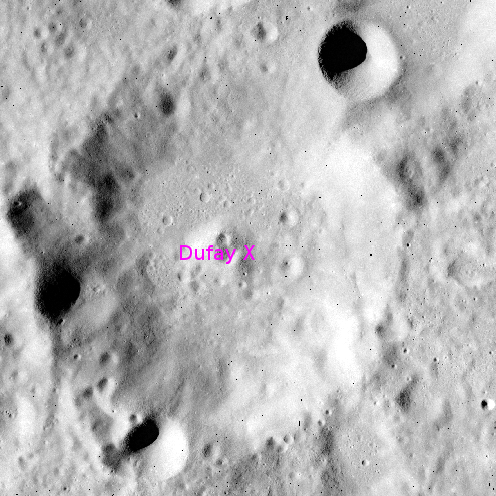Fig 1A: A cropped view of Apollo image AS16-M-0025 centered on the Dufay X crater. The spacecraft was very close to nadir when the image was taken.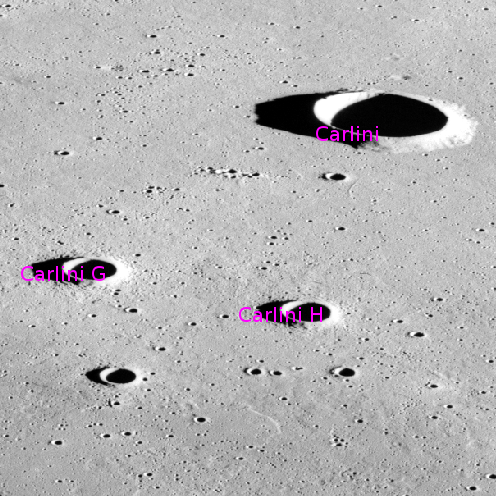Fig 2A: A cropped view of Apollo image AS15-M-1556 centered on the Carlini crater group. This photo was taken at an oblique angle.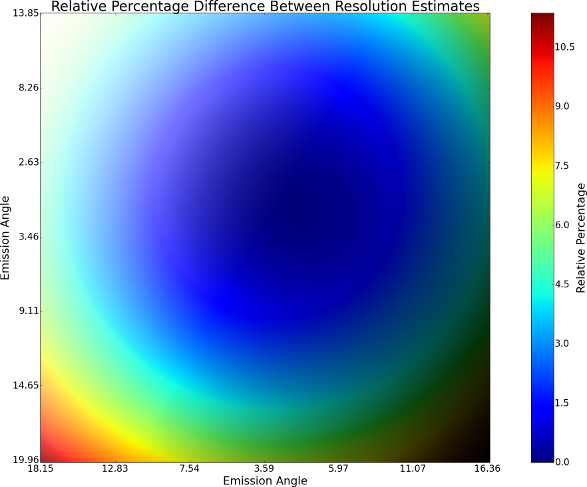Fig 1B: Relative percentage difference between resolution estimates for a nadir image (see Fig. 1A).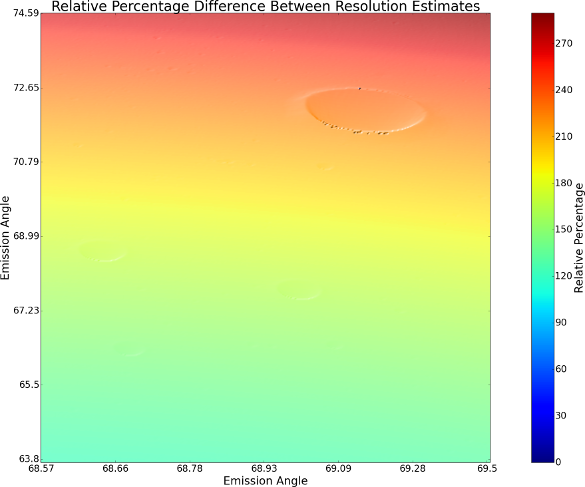Fig 2B: Relative percentage difference between resolution estimates for an oblique image (see Fig. 2A).
Handwritten notes by Orrin Thomas giving a geometric justification for this method can be found here.

## Oblique Line Resolution

The ground distance (in meters) from the top edge to the bottom edge of a pixel. This value is based on the oblique detector resolution estimate, rather than the detector resolution and is more accurate (particularly for off-nadir images).

## Oblique Pixel Resolution

The ground distance (in meters) from the left edge to the right edge of a pixel. This value is based on the oblique detector resolution estimate, rather than the detector resolution and is more accurate (particularly for off-nadir images).

## Oblique Sample Resolution

The ground distance (in meters) from the left edge to the right edge of a pixel. This value is based on the oblique detector resolution estimate, rather than the detector resolution and is more accurate (particularly for off-nadir images).

From the spacecraft, the Off Nadir is the angle between the nadir vector (subspacecraft vector) and the look vector. Every pixel in the image will result in a different Off Nadir Angle.

## PVL

Parameter Value Language (PVL) is used extensively by ISIS as a standard keyword value type language for naming and expressing data values. PVL format in ISIS is compatible with syntax used by the Planetary Data System.

For example, the keyword labels of a typical ISIS image cube is in PVL format (refer to catlab in order to view image cube labels). A parameter name (or keyword name) is used to reference the value. The value portion of an assignment statement may contain a simple value (i.e., numeric, string, date/time value), a set or a sequence of values.

Refer to the ISIS application, getkey, for one method of extracting data values from a PVL formatted file.

## Phase Angle

The phase angle is the angle between the sun and the spacecraft at a point on the surface. ISIS applications will use SPICE to compute this angle from the ellipsoid or the elevation model (e.g., local slopes) at every pixel.

Phase Angle is in degrees.

## Pixel Resolution

The ground distance in meters from the left edge to the right edge of a pixel.

## Planetary Data System

The Planetary Data System (PDS) is an archive of scientific data acquired from NASA planetary missions, astronomical observations, and laboratory measurements. By developing standards for data and archive formats and architectures for use within the archive, the PDS ensures long-term access and usability of NASA planetary data.

The PDS is organized as a federation of ten teams (nodes) geographically distributed throughout the United States. Science disciplines represented by the PDS include the Atmospheres Node, Geosciences Node, Cartography and Imaging Sciences Node, Planetary Plasma Interactions Node, Ring-Moon Systems Node, and Small Bodies Node. Two support entities include the Engineering Node and the Navigation and Ancillary Information Facility (NAIF) Node. Additional functions include a Radio Science specialist and a User-Centered Design team.

## Planetocentric Latitude

Planetocentric Latitude is the angle between the equatorial plane and a line from the center of the body.

For more details refer to the definition for Latitude Type and the interactive demonstration in the "Learning About Map Projections" on the ISIS Workshop website.

## Planetographic Latitude

Planetographic Latitude is the angle between the equatorial plane and a line that is normal (perpendicular) to the surface body.

For more details refer to the definition for Latitude Type and the interactive demonstration in the "Learning About Map Projections" on the ISIS Workshop website.

The distance from the geometric center of an ellipsoid to either of its poles.

## Polygon Thickness

The thickness of a polygon is defined as follows:

```              Thickness = Area / max(Xextent,Yextent)**2
```
A polygon's thickness will be between 0 and 1.

## Positive East Longitude

The reported longitude values for a target body increase positive to the East.

For more details refer to the definition for Longitude Direction and the interactive demonstration in the "Learning About Map Projections" on the ISIS Workshop website.

## Positive West Longitude

The reported longitude values for a target body increase positive to the West.

For more details refer to the definition for Longitude Direction and the interactive demonstration in the "Learning About Map Projections" on the ISIS Workshop website.

## Prime Meridian

Prime Meridian (W) location is the third Euler angle which is expressed as a rotation about the North Pole as a function of time. The reference frame is J2000.

## Projection X

Projection X is the x-coordinate (Easting) for a point on a geographic Cartesian coordinate system

## Projection Y

Projection Y is the y-coordinate (Northing) for a point on a geographic Cartesian coordinate system

A measurement describing the amount of electromagnetic energy emitted from an area of a planet.

The distance (vector) value between the center of and a point on a circle, sphere, ellipse or ellipsoid. Refer to qview, camstats, or campt as examples of applications that report radius value at every image pixel location.

ISIS expects and reports the radius value in Meters.

ISIS requires a radius DEM model in order to orthorectify an image (refer to spiceinit- shape model/cam2map). This is not to be confused with a topography file where the radius is subtracted from the (best fit triaxial or aeroid) shape of the target body.

## Reference Measure

Reference Measure is a fundamental component of the control network. For every point within the network, there is always one and only one associated Reference Measure (Reference=True). The Reference Measure refers to a single image and it's pixel coordinate (measure) that best represents a control point location or feature.

This specific element of the control network is exclusively used for co-registering all other overlapping and potential measures (images) to the Reference Measure.

There are control network applications that can set the Reference=True explicitly (cnetref, qnet) or 'runtime' implicitly (If reference is not set, the implicit default is the first 'valid' measure listed within a control point, refer to the pointreg application for more information).

## Reflectance

The ratio of reflected energy to incoming energy.

## Right Ascension

Right Ascension (RA) is one of two angles of the North Pole of a target body as a function of time.

## SPICE

Spacecraft & Planetary ephemerides, Instrument C-matrix and Event kernels. SPICE refers to all the information required by ISIS in order to compute and map each image pixel onto a surface with reference to spacecraft position, sun position, instrument, and mission activities. ISIS uses software (ToolKit) supplied by the Navigation and Ancillary Information Facility (NAIF) for SPICE access and kernel management (Refer to spiceinit).

SPICE is available only for mission instruments supported by ISIS (camera models).

## Sample

A column of pixels in an image, typically representing spatial information. The number of samples indicates the total width of an image in pixels.

## Sample Resolution

The ground distance (in meters) from the left edge to the right edge of a pixel.

## Scale

The map resolution measured in pixels per degree

## Serial Number

A unique identifier constructed and assigned to each individual image within a control network. The serial number (SN) is constructed by most of the control network applications "in real time" from specific instrument keywords on the image labels (Instrument keywords are populated by mission specific ingestion applications).

The serial number associated with each control measure is included as a field in the binary control network. ISIS getsn application will return the SN value for a given image filename.

## Slant Distance

The distance from the spacecraft to the point of interest on the surface of the planet.

Slant Distance is reported in kilometers.

## Slew Angle

From the spacecraft, the Slew is the angle between the boresight (i.e., center of framing camera) and the nadir vector (subspacecraft vector). The Slew angle will be a constant value across the image.

SlewAngle is in degrees.

## Solar Longitude

The Solar Longitude is the planetocentric longitude of the sun as seen from a point on a body. It is considered a seasonal angle.

Also referred to as L_sub_S.

## Spacecraft Azimuth

A clockwise angle from a point of origin to the direction of the Spacecraft.

The Spacecraft Azimuth is measured in a positive clockwise direction from a reference line. The reference line is formed by a line drawn horizontally from the origin point (pixel location) to the right side of the image. Refer to Azimuth.

## Spacecraft Position

The Spacecraft Position is the position of the spacecraft (x,y,z) in body-fixed rotating coordinate system.

Spacecraft position in body-fixed frame kilometer units.

## Special Pixels

Special Pixels are defined to distinguish valid pixels from non-valid pixels.

## SubSolar Latitude

The latitude of the subsolar point.

## SubSolar Longitude

The longitude of the subsolar point.

## SubSolar Point

The subsolar point is the target surface intercept of the line from the Sun and the target body's center. A triaxial ellipsoid is used to model the surface of the target body.

## SubSpacecraft Ground Azimuth

The Ground azimuth to the SubSpacecraft point (where the look vector from the spacecraft intercepts the target body) is obtained by taking true North (90 deg Latitude) and finding the clockwise angle to the subspacecraft latitude and longitude point.

The subspacecraft ground azimuth is complementary to the emission angle and can help to determine if two images would make a good stereo pair. If the camera position (subspacecraft ground azimuth) is the same in both images they would not offer stereo. If the camera position is slightly off between the two images, then they would make a good stereo pair

SubSpacecraft Ground Azimuth is in degrees.

## SubSpacecraft Latitude

The latitude value of the Subspacecraft Point

## SubSpacecraft Longitude

The longitude value Subspacecraft Point

## SubSpacecraft Point

The point on the surface of the target body which lies directly beneath the spacecraft.

## Subsolar Ground Azimuth

The Ground azimuth to the SubSolar point is obtained by taking true North (90 deg Latitude) and finding the clockwise angle to the subsolar latitude and longitude point.

The Subsolar Ground Azimuth is complementary to the incidence angle and gives more information about which direction the sun is coming from.

SubSolar Ground Azimuth is in degrees.

## Sun Azimuth

A clockwise angle from a point of origin to the direction of the Sun.

The Sun Azimuth is measured in a positive clockwise direction from a reference line. The reference line is formed by a line drawn horizontally from the origin point (pixel location) to the right side of the image. Refer to Azimuth.

## Target Center Distance

The distance from the spacecraft to the target body center.

Target Center Distance is in kilometers

## UTC

Coordinated Universal Time (UTC) is a system of time keeping that gives an understandable name to each instant of time. The names are formed from the calendar date and time of day. UTC format consists of year, month, day, hour, minutes and seconds.

UTC is in string format.

## Universal Coordinate

The ISIS default coordinate system

```              LatitudeType = Planetocentric
LongitudeDirection = East
LongitudeDomain = 360
```